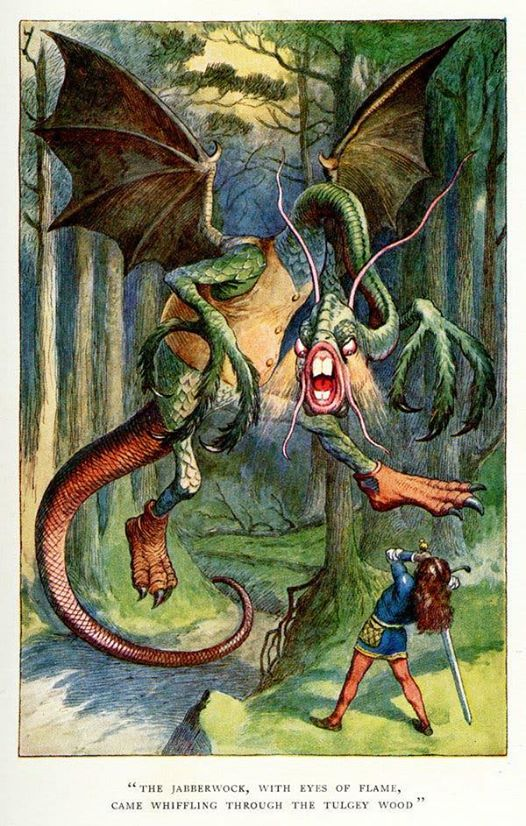# Geometry Class: The Obtuse Angle

## In geometry, which type of angle is greater than 90 degrees, but less than 180 degrees?

• A. obtuse
• B. reflex
• C. acute
• D. Nick Lachey

66% of players knew the correct answer: A., obtuse

In geometry, there are three basic types of angles. An acute angle is smaller than 90 degrees, a right angle equals 90 degrees, and an obtuse angle measures larger than 90 but less than 180 degrees.